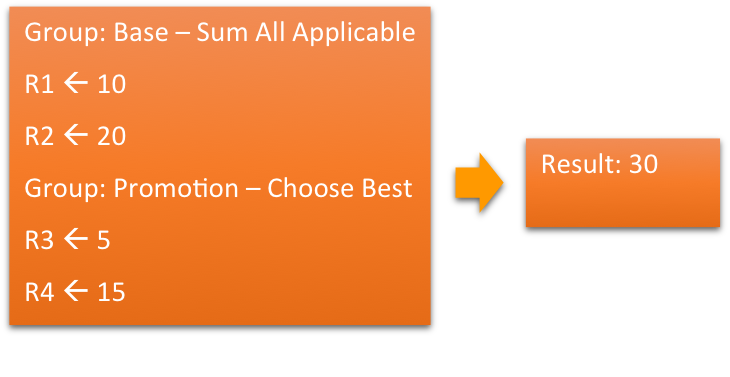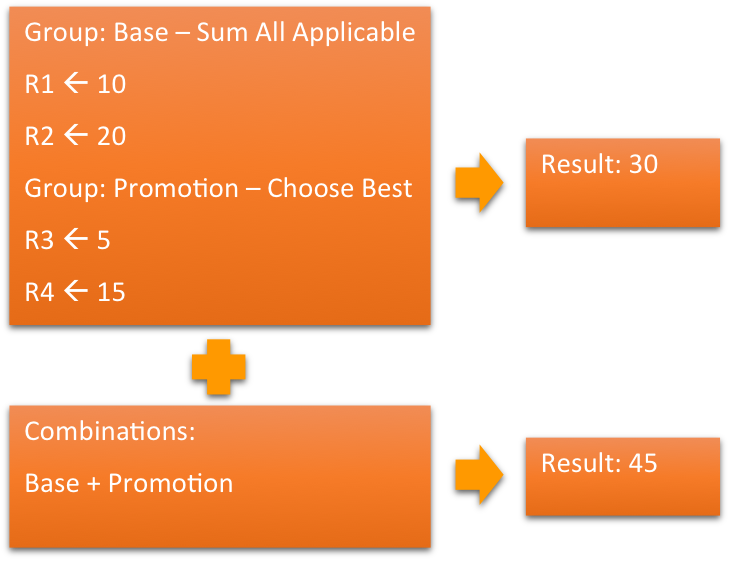# Earn Rules

Earn rules specify how members earn metrics (such as points) for each activity type. Cheetah Digital Loyalty has a purpose-built rule engine to handle common loyalty rules scenario such as layering promotional rules on top of base rules and applying only one promotion from applicable promotions.

## Rule Definition

Each row on the Rules | Earn Rules screen represents one rule. A rule has the following elements:

• Activity Types. One or more activity types as part of whose ingestion this rule is applied.
• Metric. The metric whose value is computed by this rule; note that each rule is specific to a metric.
• Calculation. The earned metric value from this rule (note that this value may combine with the result of other rules as describe below for determining the final result).
• Condition. If specified, this rule is applied only if the condition is true.
• Status. The lifecycle status of the rule including effectivity period.

### Calculation and Condition Expressions

Calculations and conditions are expressions written in Groovy language. Helper Calculation Functions encapsulate common scenarios and simplify rule authoring. Further, custom encapsulation of calculation or condition scenarios can be written as member functions. Many rule calculations may be simply accomplished as a lookup from a spreadsheet of key/value pairs.

## Grouping and Combining Rules

In Settings | Earn Rule Settings, Rule Groups and Combinations can be set up which determine how the rule engine behaves.

### Rule Groups

Rule Groups in addition to providing organization, are important semantic tools to implement common loyalty scenarios. Each rule group supports one of the following strategy for handling multiple applicable rules within a group:

• Sum all applicable rules. Results from all applicable rules within these groups are summed to calculate the result for the group.
• Use best rule result. The best result among all applicable rule results from this group is used as the group result. For accrual rules, highest value is the best.

### Rule Combinations

Rule combinations allow results from multiple rule groups to be summed or combined using other functions. A common scenario is to create a combination - promotions + base - to say that promotional rules are applied on top of base rules.

### How Final Metric is Calculated

For each rule group, a rule group result for the metric is calculated either by summing or using the best applicable rule results for the group, as per the group’s strategy. Then these group results are combined using specified combinations.

The best result from among all the results from rule groups and combination is the final calculated value for the metric.The above diagram shows a simple scenario where there are 2 rule groups, first of type ‘sum all applicable rules’, and second of type ‘use best result’. Let’s assume all the rules are applicable.

Then the result for the first group is 30, that is 10 + 20, and the result from the second group is 15, that is best of 5 and 15.

The final result then is 30, that is best of 30 and 15.

Now, let’s add a combination to this scenario, as shown in the following diagram.The result for the combination is result of group 1 + group 2, that is 30 + 15 = 45.

The final result now is 45, the best of 30, 15, and 45.

## Examples of Calculation Rules

For every PoS Purchase, give 1 point for 1\$ spent

• Activity Types: PoS Purchase
• Metric: Points
• Calculation:
``````getActivityValue('amount')
``````
• Condition: Empty

Give 15 points bonus for purchases more than \$200

• Activity Types: PoS Purchase
• Metric: Points
• Calculation: 15
• Condition:
``````getActivityValue('amount') >= 200
``````

Advanced. Give different points for items purchased. Lookup - point_lookup - defines how many points to give for every \$ spend for items in different category. The result in the lookup is stored in column point_factor. The point_factor from the lookup needs to be multiplied by the amount spent on the item.

• Activity Types: PoS Purchase
• Metric: Points
• Calculation: 15
• Condition:
``````sumActivityItems({item -> getLookupValue ('point_lookup', item.category, 'point_factor') * item.amount})
``````